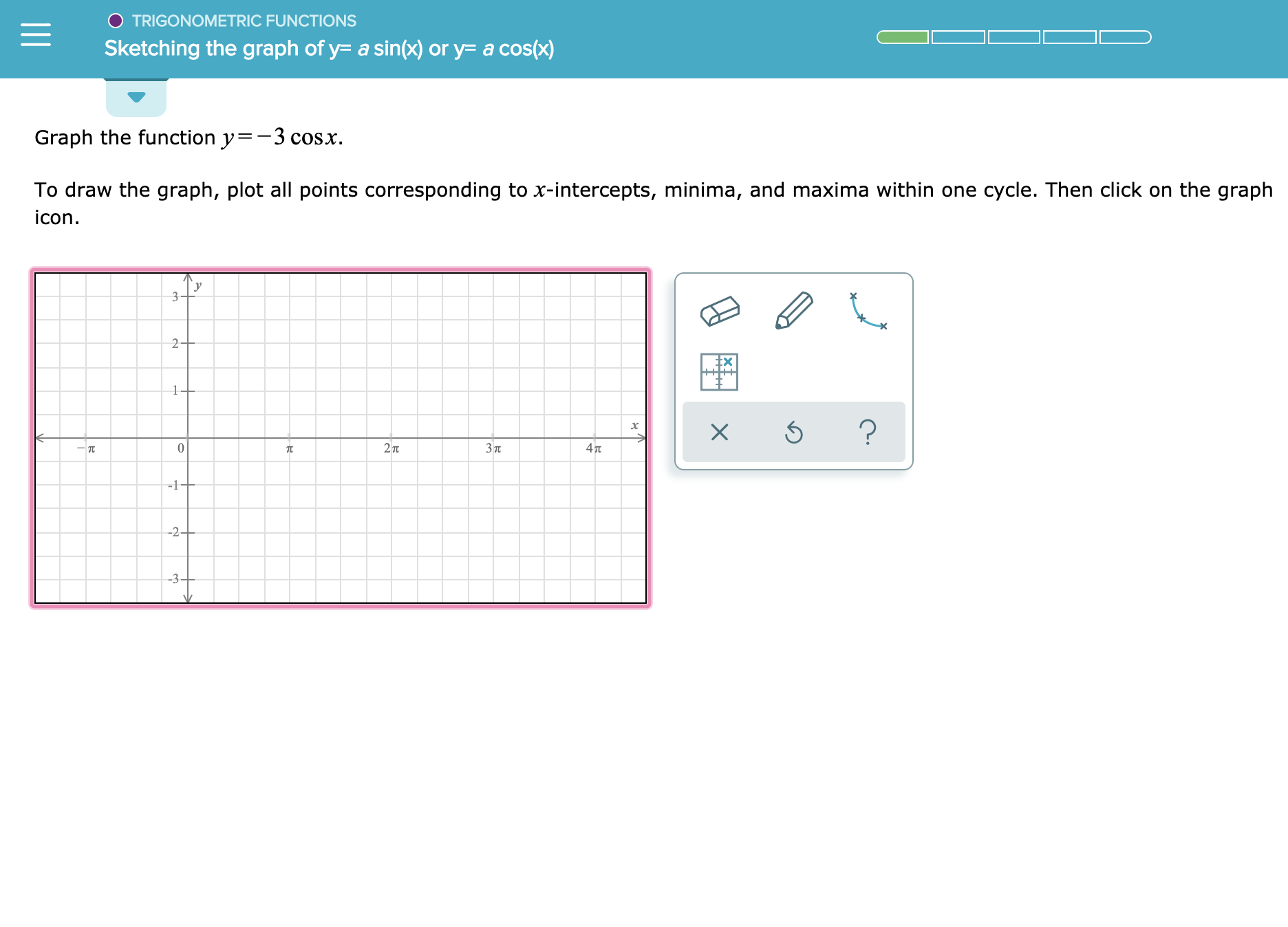# O TRIGONOMETRIC FUNCTIONS Sketching the graph of y- a sin(x) or y= a cos(x) Graph the function y-3 cosx To draw the graph, plot all points corresponding to x-intercepts, minima, and maxima within one cycle. Then click on the graph icon у 3 2+ 1+ ? 0 27 Зп 4T -1+ -2+ -3-

Question

See attachmenthelp_outlineImage TranscriptioncloseO TRIGONOMETRIC FUNCTIONS Sketching the graph of y- a sin(x) or y= a cos(x) Graph the function y-3 cosx To draw the graph, plot all points corresponding to x-intercepts, minima, and maxima within one cycle. Then click on the graph icon у 3 2+ 1+ ? 0 27 Зп 4T -1+ -2+ -3- fullscreen

### Want to see the step-by-step answer?